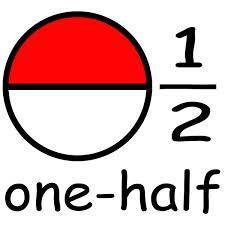What Is 11/13 As A Decimal?

Bianca da Silva answered

In order to convert 11/13 to a decimal, you will need to divide the numerator, which is 11 by the 13, which is the denominator. When you divide 11 by 13, you get 0.84615384615.Usually, you will be asked to round off this number to the nearest two decimal places. To do this, you will need to consider whether the third decimal point in above or below 5.

In the event it above 5, you will increase the second decimal by 1. If the third decimal is below 5, it falls away and you will leave the second decimal as is.

As it stands, rounding off 0.84615384615 to the second decimal place gives you the number 0.85.

If you want to learn how to convert decimals into fractions, you can find the answer here.

thanked the writer.